# Introduction

In most situations, we usually don’t have just one person accessing a database at a time. Many users can make requests to a database at the same time which can cause concurrency issues. What happens when one user writes and then another user reads from the same resource? What if both users try to write to the same resource? There are several problems we can run into when several users are using the database at the same time if we’re not careful:

• User 2 reads Table 2 (which User 1 has not updated yet) and then Table 1 (which User 1 already updated) so it reads the database in an intermediate state.

• Lost Update (Write-Write Conflict): Two users try to update the same record at the same time so one of the updates gets lost. For example:

• User 1 updates a toy’s price to be price * 2.

• User 2 updates a toy’s price to be price + 5, removing User 1’s update.

• User 1 updates a toy’s price but this gets aborted.

• User 2 reads the update before it was rolled back.

• Unrepeatable Reads (Read-Write Conflict): A user reads two different values for the same record because another user updated the record in between the two reads.

• User 1 reads in toy price.

• User 2 updates toy price to be toy price * 2 and commits

• User 1 reads in toy price again. User 1 reads in two different values for toy price because User 2’s write operation was interleaved. User 1 should be reading in the same value within one transaction. As such, User 1 will have to abort.

# Transactions

Our solution to those problems is to define a set of rules and guarantees about operations. We will do this by using transactions. A transaction1 is a sequence of multiple actions that should be executed as a single, logical, atomic unit. Transactions guarantee the ACID properties to avoid the problems discussed above:

• Atomicity: A transaction ends in two ways: it either commits or aborts. Atomicity means that either all actions in the Xact happen, or none happen.

• Consistency: If the DB starts out consistent, it ends up consistent at the end of the Xact.

• Isolation: Execution of each Xact is isolated from that of others. In reality, the DBMS will interleave actions of many Xacts and not execute each in order of one after the other. The DBMS will ensure that each Xact executes as if it ran by itself.

• Durabilty: If a Xact commits, its effects persist. The effects of a committed Xact must survive failures.

# Motivation for Concurrent Execution

It seems as though concurrency causes a lot of trouble, so why bother with it? There are two main advantages that come with implementing concurrency in our DBMS:

1. Increase in Throughput (transactions per second): If the DBMS is running on a single core, one Xact can use the CPU while another Xact is reading to or writing from the disk. If the DBMS is running on a multicore processor, we can ideally scale throughput with respect to the number of processors.

2. Decrease in Latency (response time per transaction): Multiple transactions can run at the same time, so one transaction’s latency need not be dependent on another unrelated transaction.

# Concurrency Control

In this note we will discuss how to enforce the isolation property of transactions (we will learn how the other properties are enforced in the note about recovery). To do this, we will analyze transaction schedules which show the order that operations are executed in. These operations include: Begin, Read, Write, Commit and Abort. The easiest way to ensure isolation is to run all the operations of one transaction to completion before beginning the operations of next transaction. This is called a serial schedule. For example, the following schedule is a serial schedule because $$T1$$’s operations run completely before $$T2$$ runs.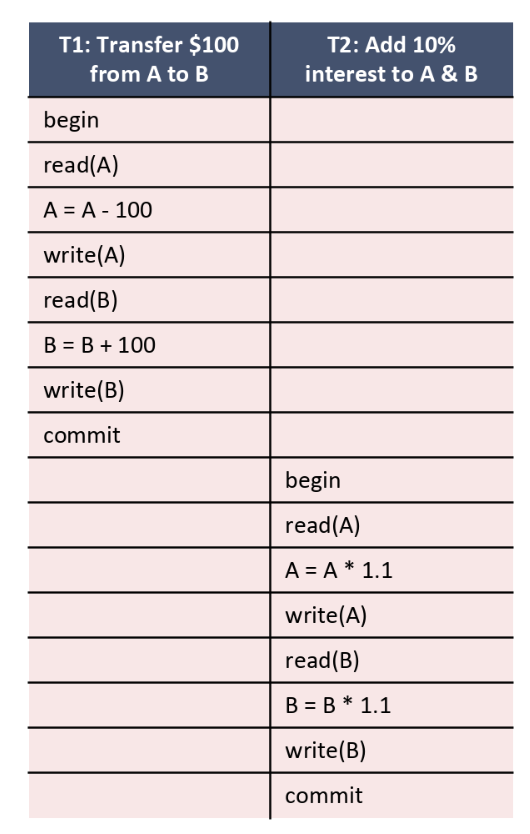The problem with these schedules, however, is that it is not efficient to wait for an entire transaction to finish before starting another one. Ideally, we want to get the same results as a serial schedule (because we know serial schedules are correct) while also getting the performance benefits of running schedules simultaneously. Basically, we are looking for a schedule that is equivalent to a serial schedule. For schedules to be equivalent they must satisfy the following three rules:

1. They involve the same transactions

2. Operations are ordered the same way within the individual transactions

3. They each leave the database in the same state

If we find a schedule whose results are equivalent to a serial schedule, we call the schedule serializable. For example, the following schedule is serializable because it is equivalent to the schedule above. You can work through the following schedule and see that resources $$A$$ and $$B$$ end up with the same value as the serial schedule above.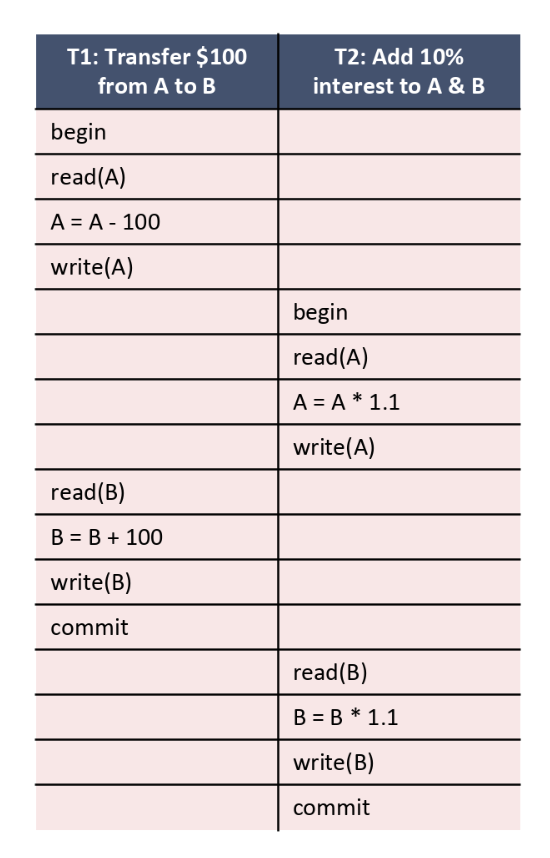## Conflict Serializability

Now the question is: how do we ensure that two schedules leave the database in the same final state without running through the entire schedule to see what the result is? We can do this by looking for conflicting operations. For two operations to conflict they must satisfy the following three rules:

1. The operations are from different transactions

2. Both operations operate on the same resource

3. At least one operation is a write

We then check if the two schedules order every pair of conflicting operations in the same way. If they do, we know for sure that the database will end up in the same final state. When two schedules order their conflicting operations in the same way the schedules are said to be conflict equivalent, which is a stronger condition than being equivalent. Now that we have a way of ensuring that two schedules leave the database in the same final state, we can check if a schedule is conflict equivalent to a serial schedule without running through the entire schedule. We call a schedule that is conflict equivalent to some serial schedule conflict serializable. Note: if a schedule $$S$$ is conflict serializable then it implies that is serializable.2.

## Conflict Dependency Graph

Now we have a way of checking if a schedule is serializable! We can check if the schedule is conflict equivalent to some serial schedule because conflict serializable implies serializable. We can check conflict serializability by building a dependency graph. Dependency graphs have the following structure:

• One node per Xact

• Edge from $$Ti$$ to $$Tj$$ if:

• an operation $$Oi$$ of $$Ti$$ conflicts with an operation $$Oj$$ of $$Tj$$

• $$Oi$$ appears earlier in the schedule than $$Oj$$

A schedule is conflict serializable if and only if its dependency graph is acyclic. So all we have to do is check if the graph is acyclic to know for sure that it is serializable!

Let’s take a look at two examples:

• The following schedule is conflict serializable and the conflict graph is acyclic. There are two conflicting operations:

• $$T1$$ reads $$A$$ and then $$T2$$ writes to $$A$$. Because of this, there will be an edge from $$T1$$ to $$T2$$.

• $$T1$$ writes to $$A$$ and then $$T2$$ reads from $$A$$. Since there already is an edge from $$T1$$ to $$T2$$, we don’t have to add the edge again.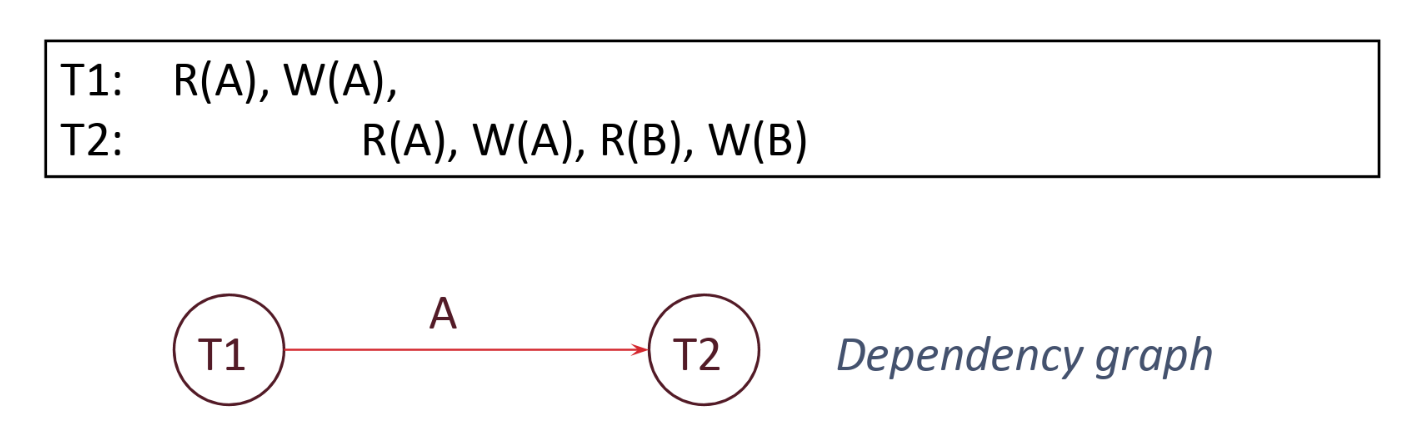• The following schedule is not conflict serializable and the conflict graph is not acyclic. Some conflicting operations:

• $$T1$$ reads $$A$$ and then $$T2$$ writes to $$A$$. Because of this, there will be an edge from $$T1$$ to $$T2$$.

• $$T2$$ writes to $$B$$ and then $$T1$$ reads $$B$$. Because of this, there will be an edge from $$T2$$ to $$T1$$.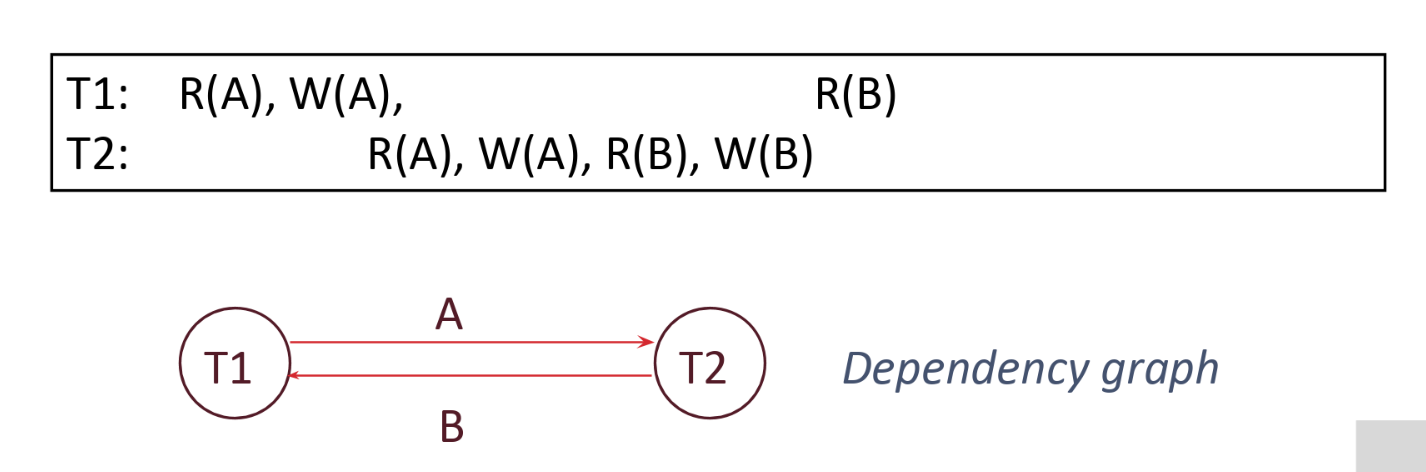## View Serializability

View serializability is an alternate way to determine overall serializability. View seriablizability has fewer false negatives with respect to conflict serializibility, meaning that it will identify more serializable schedules than conflict serializability. Schedules S1 and S2 are view equivalent if they have:

3. Same winning writes

View Serializability allows a few more schedules than conflict serializability. View serializability finds all schedules that are conflict serializable, plus a couple more that have blind writes. Blind writes are sequential writes to a data page with no interleaving reads to the page. Conflict serializability is generally preferred because it is easier and more efficient to enforce, while only missing out on a few serializable schedules with blind writes.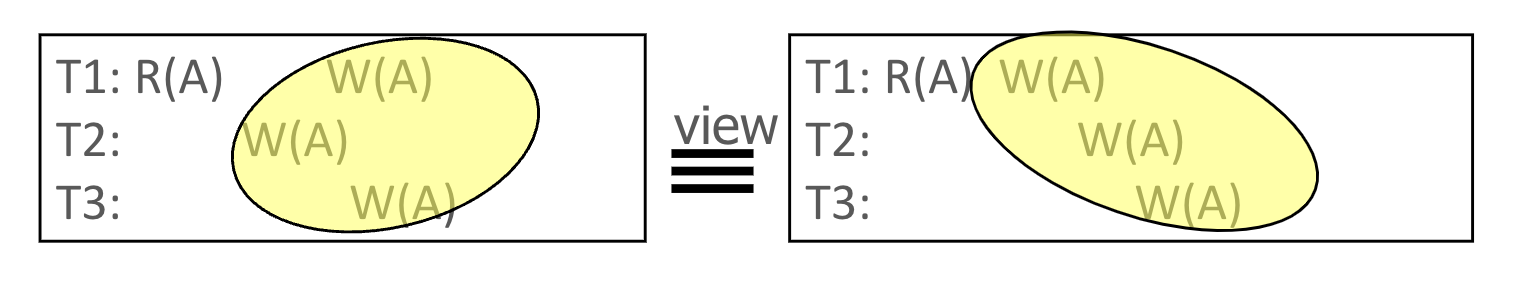# Conclusion

In this note, we removed the naive assumption we have had up until this point of only allowing one user to access a database at a time. We discussed the potential anomalies that can arise if our database does not guarantee the ACID properties. We learned how transactions are a powerful mechanism used to encapsulate a sequence of actions that should be executed as a single, logical, atomic unit. In the next note, we will discuss how to actually enforce conflict serializability for our transaction schedules.

1. We sometimes shorten transaction to Xact.

2. Not all serializable schedules are conflict serializable